# Harmonic form

(diff) ← Older revision | Latest revision (diff) | Newer revision → (diff)

An exterior differential formon a Riemannian manifoldsatisfying the equation, where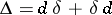is the Laplace operator corresponding to the Riemannian metric onandis the adjoint of the exterior differential. Ifhas compact support, its harmonicity is equivalent to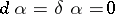. The harmonic forms of degreeonform a vector space,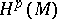, over the field. If the Riemannian manifold is compact,is finite-dimensional, being the kernel of the elliptic operator. Since a harmonic form is closed, de Rham's theorem generates a natural mapping of the spaceinto the real cohomology space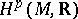of degreeof. It follows from the Hodge theorem that this mapping is an isomorphism. In particular, harmonic functions, i.e. harmonic forms of degree zero, are constant on a connected compact manifold.

Harmonic forms on a compact Riemannian manifold are invariant with respect to any connected group of isometries of this manifold; for a symmetric spacethe spacecoincides with the space of-forms which are invariant with respect to the largest connected group of isometries.

A parallel theory of harmonic forms exists for Hermitian manifolds (cf. Hermitian structure). A harmonic form on a Hermitian manifoldis a complex form lying in the kernel of the Laplace–Beltrami operator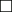(cf. Laplace–Beltrami equation). The harmonic forms of type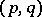constitute the space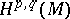over. Ifis compact,is finite-dimensional and is naturally isomorphic to the Dolbeault cohomology space. Ifis a Kähler manifold, these two definitions of harmonic forms are really identical, since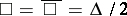. In such a caseand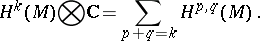Letbe the Kähler form on, letbe the operator of interior multiplication by, letbe the operator adjoint to, and let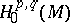be the space of primitive harmonic forms of type, i.e. formsfor which. The following equation is valid for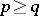and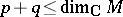: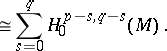For a compact Kähler manifoldthe spaceis identical with the spaceof holomorphic forms (cf. Holomorphic form) of degree. In particular,The study of harmonic functions and forms on Riemann surfaces originates with B. Riemann, whose existence theorems were fully proved at the beginning of the 20th century. The theory of harmonic forms on compact Riemannian manifolds was first presented by W.V.D. Hodge .

Various generalizations of the theory of harmonic forms were subsequently given. Let there be given a locally flat (analytic) vector bundleon a Riemannian (Hermitian) manifold, and let there be given a Euclidean (Hermitian) metric on the fibres of. By suitably generalizing the Laplace (Laplace–Beltrami) operator , , it is possible to define the spaces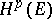(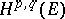) of harmonic forms with values in(cf. Differential form). Ifis compact, these spaces are finite-dimensional and isomorphic to the corresponding cohomology spaces of de Rham and Dolbeault, which can in turn be interpreted in terms of sheaf cohomology. In the case of locally flat bundles these cohomology spaces are also closely connected with the cohomology spaces of the group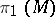. Ifis not compact, the space of square-integrable harmonic forms is isomorphic to the homology space of the complex of square-integrable forms . Ifis a domain with smooth boundary and compact closure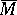in a Kähler manifold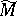, it is also possible to consider the space of harmonic forms of type, with values in an analytic vector bundleover, smooth inand continuous on. Ifis strictly pseudo-convex, this space is finite-dimensional and is isomorphic to the Dolbeault cohomology space corresponding toover.

Harmonic forms are a powerful tool in the study of the cohomology of real and complex manifolds and of cohomology spaces of discrete groups. The theory of harmonic forms yields fundamental cohomological properties of compact Kähler manifolds and, in particular, of projective algebraic varieties , , . Harmonic forms can be used to establish a connection between the curvature of a compact Riemannian manifold and the triviality of some of its cohomology groups , . Similar connections have also been obtained in complex analytic geometry ,  and in the theory of discrete transformation groups .

How to Cite This Entry:
Harmonic form. Encyclopedia of Mathematics. URL: http://encyclopediaofmath.org/index.php?title=Harmonic_form&oldid=14757
This article was adapted from an original article by A.L. Onishchik (originator), which appeared in Encyclopedia of Mathematics - ISBN 1402006098. See original article